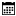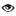s

#### Sample Programs

Murugan Andezuthu Dharmaratnam |01 April 2021 |3627

In this article, we will write an 8086 assembly language program to read a numeric single-digit ASCII character, convert it into a number and store the value in al register. Writing a program to read a number is not simple like how you could read a number using one line of code in C or C . Here you have to read the numeric characters one by one and then convert the characters into a number. In our case, it's a single-digit number so it's much simpler.

First, we will have to understand the value of numeric characters from 0 to 9. For that let's look at ASCII Character Table. Open this link ASCII Character Table. Here you can see there are 4 columns Char Dec Oct Hex. Let's focus only on two columns Char & Hex. From this table you can find the hex value of character 0 is 0x30 and the hex value of 9 is 0x39. This means when a user press number 0 on keyboard the value of the character read will be 0x30 . To convert this ASCII value to numeric we have to subtract the value of 0x30 from the ASCII value.

## Sample Code

```.model tiny
.code
org 100h

main proc near

mov ah,09h
mov dx,offset messageenternumber
int 21h

mov ah,01h  ; dos function to read a character with echo from keyboard
; result (character entered) is stored in al
int 21h     ; dos interrupt 21h

cmp al,30h  ; check if input character is less then 0,
; here we are validating user input to check if entered character is betwen 0 and 9

cmp al,39h  ; check if input character is great then 9

sub al,30h  ; subtract 30h character code of character 0 from input to get numeric value
mov bl,al   ; store value of read character in bl

mov ah,4ch      ; dos function 4ch to terminate and return to dos
mov al,00
int 21h         ; dos interrupt 21h

endp
messageenternumber db 0ah,0dh,'Please input a single digit number \$'
end main

```